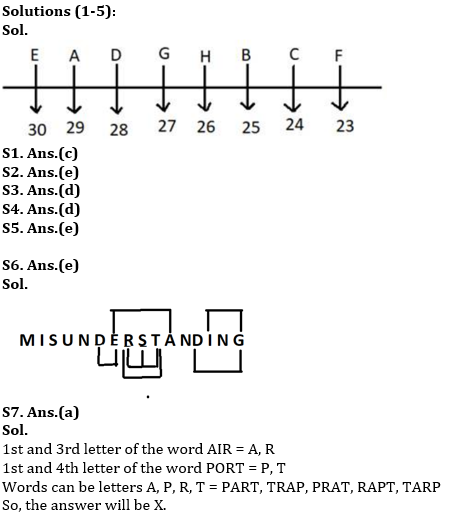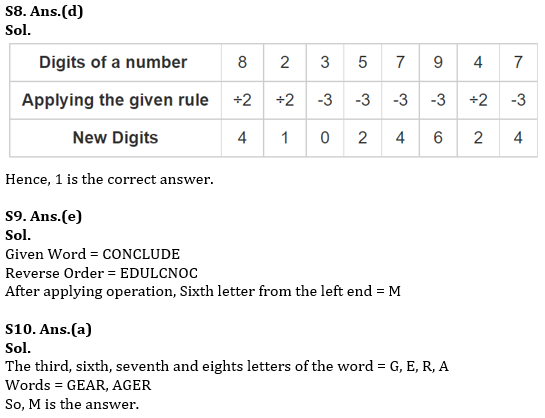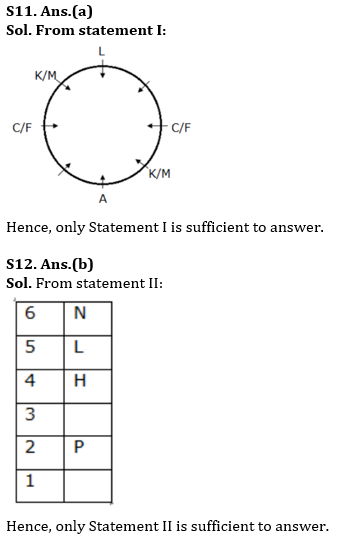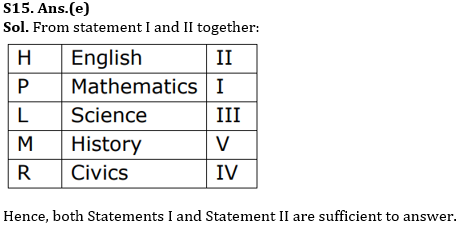Latest Banking jobs   »   RBI Assistant Vacancy 2022   »

# Reasoning Ability Quiz For RBI Assistant/ ESIC UDC Mains 2022- 01st April

Directions (1-5): Study the following information carefully and answer the questions given below.
Eight friends A, B, C, D, E, F, G and H are sitting in a row on chairs numbered 23 to 30 in ascending order from left to right and all of them are facing south. C sits on the chair no. 24. There are four persons between C and A. B and H sit adjacent to each other. G sits adjacent to neither A nor C. E never sits on an odd-numbered chair. Neither B nor G sits on chair number 26. There is only one person between F and B. D sits left of E.

Q1. Who amongst the following sits to the immediate left of E?
(a) F
(b) C
(c) A
(d) B
(e) None of these

Q2. Which of the following pairs sits at extreme ends of the row?
(a) D, C
(b) H, D
(c) G, H
(d) B, A
(e) None of these

Q3. How many persons are there between A and B?
(a) One
(b) None
(c) Two
(d) Three
(e) None of these

Q4. Four of the following five are alike in a certain way and hence from a group. Which is the one that does not belong to that group?
(a) G
(b) B
(c) F
(d) H
(e) A

Q5. Who among the following person sits on chair no. 25?
(a) H
(b) E
(c) A
(d) D
(e) None of these

Q6. How many such pairs of letters are there in the word MISUNDERSTANDING, each of which has as many letters between them in the word (both forward and backward direction) as they have between them in the English Alphabet?
(a) One
(b) Two
(c) Three
(d) Four
(e) More than four

Q7. If it is possible to make a meaningful word from 1st and 3rd letter letters of the word AIR and 1st and 4th letter of the word PORT, then which will be the second letter from the left? If more than one word will be formed, then marked as X and if no word will be formed marked it as Y.
(a) X
(b) Y
(c) R
(d) P
(e) T

Q8. If the even digits of the number 82357947 are divided by 2 and the odd digits are decreased by 3, then what will be the sum of the third digit from the left and the seventh digit from the right?
(a) 2
(b) 5
(c) 6
(d) 1
(e) 4

Q9. If in the word CONCLUDE, al the letters are written in the reverse order (i.e starting from E) and then all the vowels are changed to the next second letter and all the consonants are changed to the previous letter. After the final arrangement which of the following letter is the sixth from the left end?
(a) B
(b) C
(c) G
(d) K
(e) M

Q10. It is possible to make a meaningful word with the third, sixth, seventh and eights letters of the word “REGENERATION which of the following will be the second letter from the right end of that newly formed word? If more than one such word can be made, give M as the answer and if no such word can be made, give Y as the answer?
(a) M
(b) E
(c) Y
(d) A
(e) R

Directions (11-15): Each of the questions below consists of a question and two statements numbered I and II given below it. You have to decide whether the data given in the statements are sufficient to answer the question. Read both statement and choose the most appropriate option.

Q11. Eight persons A, C, L, K, F, H, D and M are sitting around a circular table facing centre. Three persons sit between A and L. Who sits opposite to C?
I. Only one person sits between L and F. K sits third to the right of F. K and M are not immediate neighbours. C sits third to the left of M.
II. Only one person sits between H and D. As many persons sit between M and C sit between L and H. A and K are not immediate neighbours.
(a) Only Statement I is sufficient to answer
(b) Only Statement II is sufficient to answer
(c) Either Statement I or Statement II is sufficient to answer.
(d) Neither Statement I nor Statement II is sufficient to answer.
(e) Both Statements I and Statement II are sufficient to answer.

Q12. Six persons P, Q, M, L, H and N lives in different floor. Lowermost floor is numbered 1, above floor is numbered 2, and so on. Topmost floor is numbered 6. Who lives on fifth floor?
I. P lives on even numbered floor. As many persons live below P live above L. Only one person lives between M and L.
II. H lives immediately below L, who does not live on even numbered floor. Three persons live between N and P. N lives above P. M lives below N, but not immediately below.
(a) Only Statement I is sufficient to answer
(b) Only Statement II is sufficient to answer
(c) Either Statement I or Statement II is sufficient to answer.
(d) Neither Statement I nor Statement II is sufficient to answer.
(e) Both Statements I and Statement II are sufficient to answer.

Q13. Six persons K, R, H, M, L and P attends the class in a week starting from Monday and ends on Saturday. Which of the following person attends the class on Thursday?
I. R attends the class before Wednesday. As many persons attend the class before R attends the class after M. L attends the class after M. More than three persons attend the class between L and H. K attends the class before P.
II. Two persons attend the class between M and R. H attends the class before R. As many persons attends the class before K attends the class after P.
(a) Only Statement I is sufficient to answer
(b) Only Statement II is sufficient to answer
(c) Either Statement I or Statement II is sufficient to answer.
(d) Neither Statement I nor Statement II is sufficient to answer.
(e) Both Statements I and Statement II are sufficient to answer.

Q14. Six persons M, N, R, V, S and T attends the lecture in different months January, February, March, May, June and September but not necessarily in the same order. How many persons attend the lecture between M and V?
I. R attends the lecture in a month which has maximum number of days. Three persons attend the lecture between R and T. As many persons attend the lecture before N attends the lecture after S.
II. V attends the lecture in a month which has minimum number of days. As many persons attend the lecture before V attends the lecture after T. S attends the lecture immediately before N. M attends the lecture after R.
(a) Only Statement I is sufficient to answer
(b) Only Statement II is sufficient to answer
(c) Either Statement I or Statement II is sufficient to answer.
(d) Neither Statement I nor Statement II is sufficient to answer.
(e) Both Statements I and Statement II are sufficient to answer.

Q15. Five persons H, P, L, M and R likes five different subject English, Science, Mathematics, Civics and History and they are studying in different class I, II, III, IV and V. All the above information is not necessarily in the same order. Who studies in class I?
I. M neither likes Science nor like English. H neither studies in class I or IV. P likes Mathematics. L studies in class III. R does not like English. The one who likes English studies in class II.
II. Neither P nor M studies in class IV. The one who likes History studies in class V. L does not like Civics.
(a) Only Statement I is sufficient to answer
(b) Only Statement II is sufficient to answer
(c) Either Statement I or Statement II is sufficient to answer.
(d) Neither Statement I nor Statement II is sufficient to answer.
(e) Both Statements I and Statement II are sufficient to answer.

Solutions